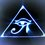# Rewriting Equations of Tangents

A tangent is commonly written in the form y=mx+b

Generally to find the equation of the tangent of a function at a given point you find the derivative, or the slope of the function at the given point, and then you substitute x and y values to solve for b. But by writing the equation differently you can skip the last step.

Instead this method states that once you have calculated the derivative of the function you approach the problem by solving for the horizontal and vertical shift.

Thus given f(x), the equation of the tangent to the function f(x) is:

y=m(x-x)+(f(x))

heres a quick example.

f(x)= x^2-6x+17

dy/dx = 2x-6 (used the power rule for calculating derivatives, this is "m" in y=mx+b)

thus the equation of the tangent:

y=(2x-6)(x-x)+(x^2 - 6x+17)

this solves for the equations of all tangents to the function f(x).

substituting a value for x into (2x-6) solves for the slope at that given x value.

Substituting the same value for x into the second x only * of (x-x) will apply a horizontal shift. The first x remains variable.

and substituting x into (x^2 - 6x+17) will apply the vertical shift.Note by Brody Acquilano
6 years, 4 months ago

This discussion board is a place to discuss our Daily Challenges and the math and science related to those challenges. Explanations are more than just a solution — they should explain the steps and thinking strategies that you used to obtain the solution. Comments should further the discussion of math and science.

When posting on Brilliant:

• Use the emojis to react to an explanation, whether you're congratulating a job well done , or just really confused .
• Ask specific questions about the challenge or the steps in somebody's explanation. Well-posed questions can add a lot to the discussion, but posting "I don't understand!" doesn't help anyone.
• Try to contribute something new to the discussion, whether it is an extension, generalization or other idea related to the challenge.

MarkdownAppears as
*italics* or _italics_ italics
**bold** or __bold__ bold
- bulleted- list
• bulleted
• list
1. numbered2. list
1. numbered
2. list
Note: you must add a full line of space before and after lists for them to show up correctly
paragraph 1paragraph 2

paragraph 1

paragraph 2

[example link](https://brilliant.org)example link
> This is a quote
This is a quote
    # I indented these lines
# 4 spaces, and now they show
# up as a code block.

print "hello world"
# I indented these lines
# 4 spaces, and now they show
# up as a code block.

print "hello world"
MathAppears as
Remember to wrap math in $$ ... $$ or $ ... $ to ensure proper formatting.
2 \times 3 $2 \times 3$
2^{34} $2^{34}$
a_{i-1} $a_{i-1}$
\frac{2}{3} $\frac{2}{3}$
\sqrt{2} $\sqrt{2}$
\sum_{i=1}^3 $\sum_{i=1}^3$
\sin \theta $\sin \theta$
\boxed{123} $\boxed{123}$